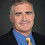## Wednesday, August 30, 2023

### What Makes a Constructive Proof?

In this weblog, we've used constructive in different ways. Often we talk about constructive as something we can create in polynomial time, like an expander. But how about constructive as in logic, when you don't get to assume the "excluded middle" where you get to assume some statement is either true or false?

The simplest well-known example is the theorem: There exists irrational $$a$$ and $$b$$ such that $$a^b$$ is rational.

1. $$\sqrt{2}^\sqrt{2}$$ is rational. Let $$a = b = \sqrt{2}$$.
2. $$\sqrt{2}^\sqrt{2}$$ is irrational. Let $$a = \sqrt{2}^\sqrt{2}$$ and $$b = \sqrt{2}$$.
You don't know which $$a$$ is correct. You just know it exists. (A far more complicated argument shows $$\sqrt{2}^\sqrt{2}$$ is in fact irrational.)

When I teach intro theory, my first proof that there are non-computable sets is by claiming the computable sets are countable but their are an uncountable number of sets over $$\Sigma^*$$ so there must be an non-computable sets. I claim this is a non-constructive proof because I didn't give you the set and do an aside on constructive proofs using the example above. But that's not correct--the proof that there are uncountable number of sets over $$\Sigma^*$$ is a constructive diagonalization. Give me an enumeration of the computable sets and I can easily construct a set not on that list.

In complexity, a well-known non-constructive theorem is by Kannan, showing that $$\Sigma^P_2$$ does not have $$n^2$$-size circuits.

1. SAT doesn't have n2-size circuits. Since SAT is in Σ2 we are done.
2. SAT has n2-size circuits. Then by Karp-Lipton Σ4 = Σ2 so L is in Σ2 and we are done.
Jin-Yi Cai and Osamu Watanabe, and independently Sunny Daniels, gave a constructive $$\Sigma^2_P$$ machine and thus a single language in $$\Sigma^P_2$$ that doesn't have $$n^2$$-size circuits. But it is not a constructive proof, as the argument the machine works requires the two cases as to whether SAT has small circuits. As far as I know, a true constructive proof of Kannan's theorem remains open.

I have no problem with non-constructive proofs—I'm in a firm believer in $$P\vee\neg P$$. But if you do talk about constructivity be sure and use it appropriately.

1.Your root 2 example has at least one typo.

1.I can't find the typo. Can you be more "constructive"?

2.Are you saying that the first proof, the one with all of the sqrt(2)s in it, is constructive as a proof by cases?

1.No, the first proof is not constructive as it depends on assuming sqrt(2)^sqrt(2) is either rational or irrational

3.Why is (sqrt(2)^sqrt(2))^sqrt(2) rational???

1.(sqrt(2)^sqrt(2)) ^ sqrt(2) = sqrt(2)^(sqrt(2) * sqrt(2)) = sqrt(2)^2 = 2

Repeated exponentiation means the exponents get multiplied.

4.In number theory, there are some examples of theorems proven by cases, giving a proof if the Riemann Hypothesis holds, and another if the Riemann Hypothesis fails. Some are mentioned in wikipedia like Nicolas' theorem. I've always wondered what constructive mathematicians thought of those.

5.I think the L in the non-constructive theorem by Kannan refers to a language in sigma_4. As stated it can easily be misunderstood as the class L. The definition of L was given in the post "Sixteen Years in the Making " (August'14) but did not survive the copy+paste.

In general, keep up the great work. This blog is awesome!

6.Haven't there been some more recent (last 5 years maybe?) circuit lower-bound results whose proofs are non-constructive in the sense of explicitly using the law of the excluded middle? I feel like I've seen at least one such paper, but unfortunately, I can't recall any more details.

7.This post reminds me of that great non-constructive joke.

8.To some fuel to the fire, constructivist don't agree on what it exactly means. See "Varieties of Constructive Mathematics".

And you can always state classical theorems in a construction way using Godel's translation.

not (not A and not B)

which indicates that essentially they are interpreting or as having a new meaning.

9.It seems that Daniels's proof can be presented in a constructive way. He defined languages L_k for any k and the description of the \Sigma^p_2 machines that decide L_k are computable in polynomial time in k. So we can write the statement B:="for every k, L_k is not in size(n^k)" as a \Pi_2 sentence in the language of arithmetic as follows:

B:=For every k and every c, there is a natural number n such that any circuit of size at most cn^k+c with input length n does not decide L_k correctly on inputs of length n.

As the property "any circuit of size at most cn^k+c with input length n does not decide L_k correctly on inputs of length n" is a primitive recursive property (it is actually a bounded formula in the language of Buss's bounded arithmetic) B is a \Pi_2 sentence. Daniels's proof can be formalized in Peano arithmetic and as it is known that Peano arithmetic is \Pi_2 conservative over Heyting arithmetic we get that Heyting arithmetic can prove B which implies the existence of a constructive proof of Daniels's result. Here is the wiki link about \Pi_2 conservativity of Peano arithmetic over Heyting arithmetic:
https://en.wikipedia.org/wiki/Friedman_translation